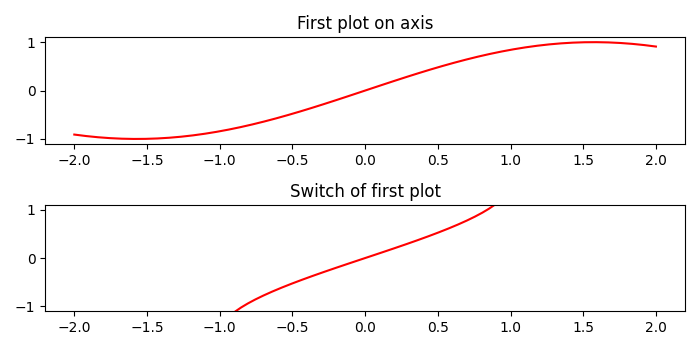# How to switch axes in Matplotlib?

To switch axes in matplotlib, we can create a figure and add two subplots using subplots() method. Plot curves, extract x and y data, and set these data in a second plotted curve.

## Steps

• Create x and y data points using numpy.

• Create a figure and add a set of two subplots.

• Set the title of the plot on both the axes.

• Plot x and y data points using plot() method.

• Extract the x and y data points using get_xdata and get_ydata.

• To switch the axes of the plot, set x_data and y_data of the axis 1 curve to axis 2 curve.

• To display the figure, use show() method.

## Example

import numpy as np
from matplotlib import pyplot as plt
plt.rcParams["figure.figsize"] = [7.00, 3.50]
plt.rcParams["figure.autolayout"] = True
x = np.linspace(-2, 2, 50)
y = np.sin(x)
f, axes = plt.subplots(2)
axes.set_title("First plot on axis")
curve, = axes.plot(x, y, c='r')
newx = curve.get_xdata()
newy = curve.get_ydata()
axes.set_title("Switch of first plot")
curve2, = axes.plot(x, y, c='r')
curve2.set_xdata(newy)
curve2.set_ydata(newx)
plt.show()

## Output